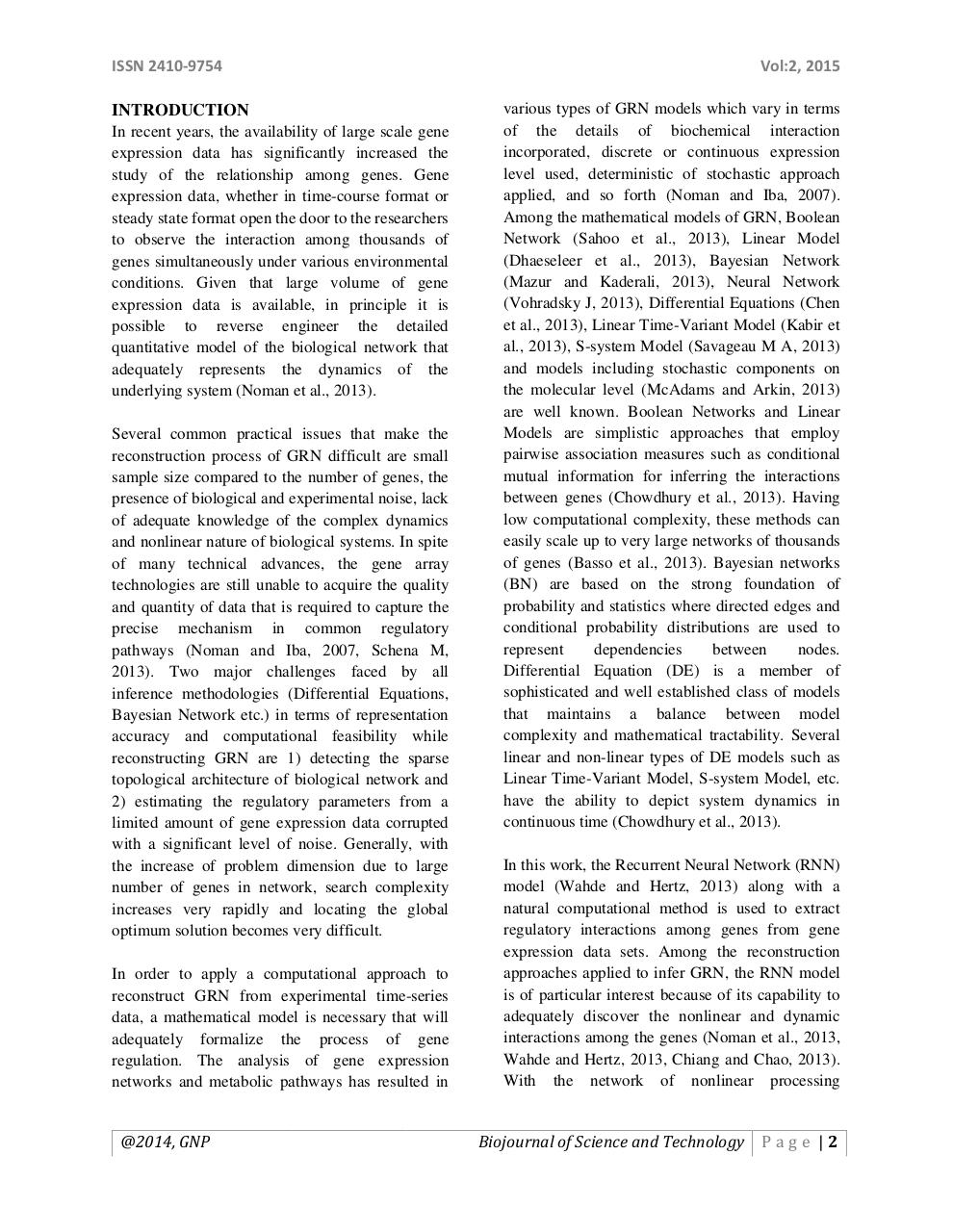# m140007.pdfPage 1 2 3 4 5 6 7 8 9 10 11

#### Text preview

ISSN 2410-9754
INTRODUCTION
In recent years, the availability of large scale gene
expression data has significantly increased the
study of the relationship among genes. Gene
expression data, whether in time-course format or
steady state format open the door to the researchers
to observe the interaction among thousands of
genes simultaneously under various environmental
conditions. Given that large volume of gene
expression data is available, in principle it is
possible to reverse engineer the detailed
quantitative model of the biological network that
adequately represents the dynamics of the
underlying system (Noman et al., 2013).
Several common practical issues that make the
reconstruction process of GRN difficult are small
sample size compared to the number of genes, the
presence of biological and experimental noise, lack
of adequate knowledge of the complex dynamics
and nonlinear nature of biological systems. In spite
of many technical advances, the gene array
technologies are still unable to acquire the quality
and quantity of data that is required to capture the
precise mechanism in common regulatory
pathways (Noman and Iba, 2007, Schena M,
2013). Two major challenges faced by all
inference methodologies (Differential Equations,
Bayesian Network etc.) in terms of representation
accuracy and computational feasibility while
reconstructing GRN are 1) detecting the sparse
topological architecture of biological network and
2) estimating the regulatory parameters from a
limited amount of gene expression data corrupted
with a significant level of noise. Generally, with
the increase of problem dimension due to large
number of genes in network, search complexity
increases very rapidly and locating the global
optimum solution becomes very difficult.
In order to apply a computational approach to
reconstruct GRN from experimental time-series
data, a mathematical model is necessary that will
adequately formalize the process of gene
regulation. The analysis of gene expression
networks and metabolic pathways has resulted in

@2014, GNP

Vol:2, 2015
various types of GRN models which vary in terms
of the details of biochemical interaction
incorporated, discrete or continuous expression
level used, deterministic of stochastic approach
applied, and so forth (Noman and Iba, 2007).
Among the mathematical models of GRN, Boolean
Network (Sahoo et al., 2013), Linear Model
(Dhaeseleer et al., 2013), Bayesian Network
(Mazur and Kaderali, 2013), Neural Network
(Vohradsky J, 2013), Differential Equations (Chen
et al., 2013), Linear Time-Variant Model (Kabir et
al., 2013), S-system Model (Savageau M A, 2013)
and models including stochastic components on
the molecular level (McAdams and Arkin, 2013)
are well known. Boolean Networks and Linear
Models are simplistic approaches that employ
pairwise association measures such as conditional
mutual information for inferring the interactions
between genes (Chowdhury et al., 2013). Having
low computational complexity, these methods can
easily scale up to very large networks of thousands
of genes (Basso et al., 2013). Bayesian networks
(BN) are based on the strong foundation of
probability and statistics where directed edges and
conditional probability distributions are used to
represent
dependencies
between
nodes.
Differential Equation (DE) is a member of
sophisticated and well established class of models
that maintains a balance between model
complexity and mathematical tractability. Several
linear and non-linear types of DE models such as
Linear Time-Variant Model, S-system Model, etc.
have the ability to depict system dynamics in
continuous time (Chowdhury et al., 2013).
In this work, the Recurrent Neural Network (RNN)
model (Wahde and Hertz, 2013) along with a
natural computational method is used to extract
regulatory interactions among genes from gene
expression data sets. Among the reconstruction
approaches applied to infer GRN, the RNN model
is of particular interest because of its capability to
adequately discover the nonlinear and dynamic
interactions among the genes (Noman et al., 2013,
Wahde and Hertz, 2013, Chiang and Chao, 2013).
With the network of nonlinear processing

Biojournal of Science and Technology P a g e | 2